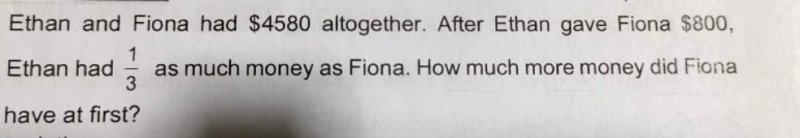# QuestionPls help.thank you

Total amount of money that both have still remains the same = \$4580

Amount Ethan has after giving = 4580/4 = 1145

Amount Ethan has originally = 1145 + 800 = \$1945
Amount Fiona has originally = (1145×3) – 800 = 2635

Amount Fiona has more than Ethan originally = 2635 -1945 = 690

0 Replies 0 Likes

At the end,

Fiona    [u][u][u]     }

}     4580  (This total has no change at first or the end)

Ethan   [u]              }

4u = 4580 => u = 1145

At first Fiona has 3u-800 = 3(1145) – 800 = 2635

At first Ethan has  4580-2635 = 1945

Hence at first, Fiona has (2635-1945) = \$690 more than Ethan.

0 Replies 1 Like
```The key observation in this type of problem is this:
The total amount of money did not change.

So at the end:
Ethan: [u]          (1/3 of Fiona)
Fiona: [u][u][u]

That means 4u = 4580 and u = 4580/4 = 1145.
So at first:
Fiona had 3 x 1145 - 800 = \$2635
Ethan had     1145 + 800 = \$1945
Fiona had 2635 - 1945 = \$690 more at first.```
```
CHECK
Ethan               Fiona
Start      1945=4580-2635      2635
End        1145=1945-800       3435=2635+800

Ratio     3435/1145 = 3

```
0 Replies 1 Like